# MATH

 ADD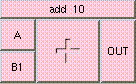Click box to see full description ```This is the addition function. The result is A + B + .... A and B may be scalar, vectors, or colors. The result will be a scalar if both A and B are scalars. The result will be a vector if either A or B is a vector. The result will be a color if either A or B is a color. A type mis-match will result from the addition of vectors and colors. ```
 SUBTRACT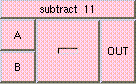Click box to see full description ```This is the subtraction function. The result is A - B. A and B can be scalars, vectors, or colors. The result will be a scalar if both A and B are scalars. The result will be a vector if either A and B are vectors. The result will be a color if either A and B are colors. A type mis-match will result from the subtraction of vectors and colors. ```
 MULTIPLY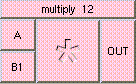Click box to see full description ```This is the multiplication function. The result is A * B. A and B can be scalars, vectors, or colors. The result will be a scalar if both A and B are scalars. The result will be a vector if either A or B is a vector. The result will be a color if either A or B is a color. A type mis-match will result from the multiplication of points and colors. ```
 DIVIDE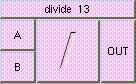Click box to see full description ```This is the division function. The result is the quotient of A / B. A and B may be scalar, vector, or colors. The result will be a scalar if both A and B are scalars. The result will be a vector if either A and B are vectors. The result will be a color if either A and B are colors. A type mis-match will result from the division of colors and vectors. ```
 ABS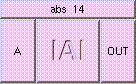Click box to see full description ```This function returns the absolute value of A: |A| ```
 SIGN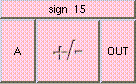Click box to see full description ```This function returns either +1 or -1 based on the sign of the input. ```
 NEGATE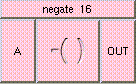Click box to see full description ```This function returns -A, the negative of input A. ```
 MODULUS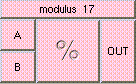Click box to see full description ```This function returns the remainder of A divided by B. ```
 REPEAT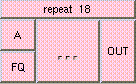Click box to see full description ```This is function returns mod(A*FQ, 1). This is the percentage of 1, which that frequency of A represents. ```
 SHIFT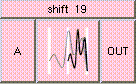Click box to see full description ```The result is (A+1)/2. This shifts the range of A from [-1,1] to [0,1]. Inputs should lie in the range [-1,1]. ```
 CONTRAST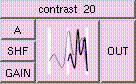Click box to see full description ```This function can increase or decrease the contrast of A. If SHF is negative and GAIN is > 1 this will increase the contrast. If SHF is positive and GAIN is < 1 the contrast will be decreased. The result will be the same data type as A. ```
 INVERSE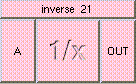Click box to see full description ```This function returns the inverse of A: 1/A. ```
 REVERSE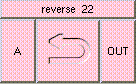Click box to see full description ```Result is 1-A. This reverses the direction of A. Inputs should lie in the range [0,1]. Vectors should be normalized. ```
 AVERAGE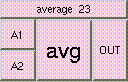Click box to see full description ```This is the average function. The result is the average value of all the inputs. The inputs may be scalars, vectors, or colors, but all inputs must be of the same type. ```
 FACTORIAL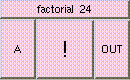Click box to see full description ```Returns the factorial of A. OUT = 1 * 2 * 3 .. A ```
 RANDOM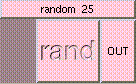Click box to see full description ```The result is a random scalar in the range [0,1]. ```
 COMPLEMENT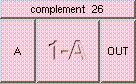Click box to see full description ```Result is 1-A. This is the complement of A. A may be a float, point or color. The result will be the same data type. A should lie in the range [0,1]. Vectors should be normalized. ```
 DIFFERENCE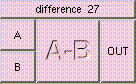Click box to see full description ```This returns the difference of A and B. The result is A - AB. A and B may be floats, colors or points, but they must be the same type. ```
 INTERSECTION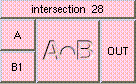Click box to see full description ```The result is A * B. This is the intersection of A and B. A and B can be floats, points or colors, but they must be the same data type. Output will be the same data type. ```
 UNION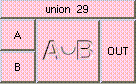Click box to see full description ```The result is A + B - (A * B). This is the union of A and B. A and B may be floats, points or colors, but they must be the same data type. Output will be the same data type. ```
 CUBE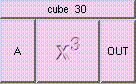Click box to see full description ```This function returns A cubed. ```
 POWER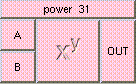Click box to see full description ```Result of this function is A raised to the power of B; Example: pow(2,3.0) = 2*2*2 = 8; Example: pow(4,0.5) = sqrt(4) = 2; ```
 SQRT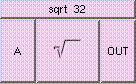Click box to see full description ```This function returns the square root of A. ```
 SQUARE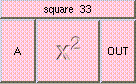Click box to see full description ```This function returns A squared. ```
 INVERSESQRT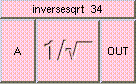Click box to see full description ```This function returns the inverse of the square root of A. ```
 E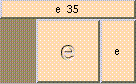Click box to see full description ```This is the constant e ~ 2.718281828459 e denotes the unquie positive real number such that log(e) = 1. ```
 EXP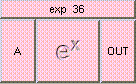Click box to see full description ```The result is e raised to the power of A. ```
 EXPM1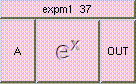Click box to see full description ```The result is e raised to the power of A minus 1.0. ```
 GAMMA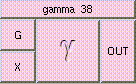Click box to see full description ```This function returns X raised to 1/G. ```
 LOG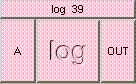Click box to see full description ```The result is the natural logarithm of A; ```
 LOG10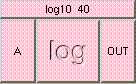Click box to see full description ```The result is the natural logarithm to base 10 of A; ```
 LOG1P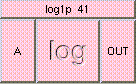Click box to see full description ```The result is the natural logarithm of (1+A); ```
 PI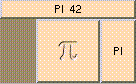Click box to see full description ```This is the constant PI ~ 3.1415927 ```
 PI2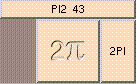Click box to see full description ```This is the constant 2*PI ~ 6.2831853 ```
 HALFPI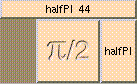Click box to see full description ```This is the constant PI/2 ~ 1.5707963 ```
 SINE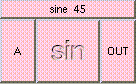Click box to see full description ```This function returns a float between [-1,1], the sine of angle A. ```
 ARCSINE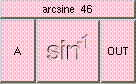Click box to see full description ```This function returns a scalar between [-PI/2,PI/2], the arcsine of A. Arcsine is the inverse of the sine function where the asin(x) = y if and only if sin(y) = x. ```
 COSINE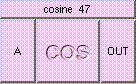Click box to see full description ```This function returns a scalar between [-1,1], the cosine of angle A. ```
 ARCCOSINE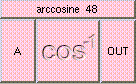Click box to see full description ```This function returns a scalar between [0,PI], the arccosine of A. Arccosine is the inverse of the cosine function where the acos(x) = y if and only if cos(y) = x. ```
 TANGENT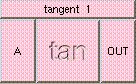Click box to see full description ```This function returns a scalar which runs to infinity as the angle A approachs PI/2, the tangent of angle A. ```
 ARCTANGENT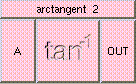Click box to see full description ```This fucntion returns a scalar between [-PI/2,PI/2], the arctangent of A. Arctangent is the inverse of the tangent function where the atan(x) = y if and only if tan(y) = x. ```
 ARCTANGENT2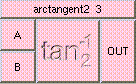Click box to see full description ```This function returns a scalar between [-PI,PI]. The sign of the inputs determines which quadrant the result will be. Arctangent is the inverse of the tangent function. atan2(b,a) = y/x if and only if tan(y/x) = b/a. ```
 RADIUS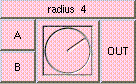Click box to see full description ```This function returns the radius of a circle inscribed in the square defined by A and B. For best results A and B should be perpendicular. Such as u and v or the lengths of two perpendicular vectors. ```
 DEG2RAD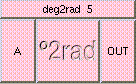Click box to see full description ```This function converts degrees to radians. It returns the input scalar, A in radians. ```
 RAD2DEG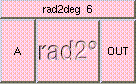Click box to see full description ```This function converts radians to degrees. It returns the input scalar, A in degrees. ```
 MIN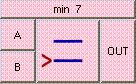Click box to see full description ```This function returns the minimum of the input values. The value returned will be of the same data type as the inputs. ```
 MAX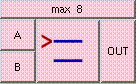Click box to see full description ```This function returns the maximum of the input values. The value returned will be of the same data type as the inputs. ```
 CEIL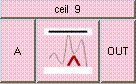Click box to see full description ```This function returns the smallest integer greater than or equal to A. ```
 FLOOR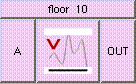Click box to see full description ```This function returns the largest integer less than or equal to A. ```
 ROUND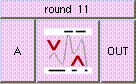Click box to see full description ```This function returns the closest integer A. ```
 CLAMP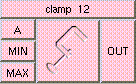Click box to see full description ```This function clamps A to the range [MIN, MAX]. Returns MIN if A < MIN or MAX if A > MAX otherwise it returns A. The data type returned will be the same type as the inputs. All inputs must be of the same type. ```
 MOTIONDIR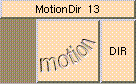Click box to see full description ```This returns the vector describing the direction of the motion. It is used in calculating motion blur. If there is no motion a null vector is returned. ```
 DUDV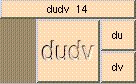Click box to see full description ```This vector represents the rate of change in the surface with respect to u and v. A vector is returned only for free-form surface objects, for polygonal objects the vector is null. ```